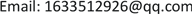﻿ (r, s)熵的稳定性的研究 Study on the Stability of (r, s) Entropy

Applied Physics
Vol. 09  No. 09 ( 2019 ), Article ID: 32372 , 7 pages
10.12677/APP.2019.99047

Study on the Stability of (r, s) Entropy

Tiantian Wang, Jiamei Wang, Wanqing Li

School of Mathematics & Physics, Anhui University of Technology, Ma’anshan AnhuiReceived: Sep. 6th, 2019; accepted: Sep. 20th, 2019; published: Sep. 27th, 2019ABSTRACT

In this paper, the stability of Tsallis entropy in reference  is generalized, and the compact upper bound of (r, s) entropy in classical cases is established by using the probabilistic coupling method, and the upper bound of the quantum condition is given in the same way. These results give some quantitative characterizations of uniform continuity and stability of entropy.

Keywords:(r, s) Entropy, Trace Distance, Probability Distribution, Density Operate

(r, s)熵的稳定性的研究1. 引言

1982年，Lesche对Renyi熵研究了这个问题，他认为Renyi r-熵对于 $r\ne 1$ 是不稳定的(或者说性质是不强的)，用数学语言来说，它们对于迹距离不是一致连续的。2002年，安倍再次讨论了Lesche型的稳定性问题 ，他证明了Tsallis熵虽然从数学角度来说与Renyi熵无关，但对于有限系统来说是稳定的。此外，Curado and Nobre ，and Abe et al.  证明了某些广义熵的稳定性。

$\frac{\Phi \left(p\right)-\Phi \left(q\right)}{{\Phi }_{m}}\le \epsilon$

2. 经典情形

${p}_{X}=\left\{{p}_{X}\left(i\right):i=1,2,\cdots ,m\right\}$

${H}_{r}^{s}\left({p}_{X}\right)=\frac{1}{\left(1-r\right)s}\left({\left(\underset{i=1}{\overset{m}{\sum }}{p}_{X}^{r}\left(i\right)\right)}^{s}-1\right)$ (1)

$H\left({p}_{X}\right)=-\underset{i}{\sum }{p}_{X}\left(i\right)\mathrm{log}{p}_{X}\left(i\right)$

${H}_{r,m}^{s}=\frac{{m}^{\left(1-r\right)s}-1}{\left(1-r\right)s}$ (2)

${H}_{r}^{s}\left({p}_{XY}\right)=\frac{1}{\left(1-r\right)s}\left({\left(\underset{i,j=1}{\overset{m}{\sum }}{p}_{XY}{}^{r}\left(i,j\right)\right)}^{s}-1\right)$

${p}_{X}^{r}\left(i\right)={\left(\underset{j}{\sum }{p}_{XY}\left(i,j\right)\right)}^{r}\ge \underset{j}{\sum }{p}_{XY}^{r}\left(i,j\right)$

${\left(\underset{i=1}{\overset{m}{\sum }}{p}_{X}^{r}\left(i\right)\right)}^{s}\ge {\left(\underset{i,j}{\overset{m}{\sum }}{p}_{XY}^{r}\left(i,j\right)\right)}^{s}$ (3)

$1-r<0$，则 ${H}_{r}^{s}\left({p}_{X}\right)\le {H}_{r}^{s}\left({p}_{XY}\right)$

${‖{p}_{X}-{p}_{Y}‖}_{K}=\frac{1}{2}\underset{i=1}{\overset{m}{\sum }}|{p}_{X}\left(i\right)-{p}_{Y}\left(i\right)|$ (4)

${‖{p}_{X}-{p}_{Y}‖}_{K}=\underset{A}{\mathrm{sup}}|\underset{i\in A}{\sum }{p}_{X}\left(i\right)-\underset{i\in A}{\sum }{p}_{Y}\left(i\right)|$

$|{H}_{r}^{s}\left({p}_{X}\right)-{H}_{r}^{s}\left({p}_{Y}\right)|\le {H}_{r}^{s}\left(\epsilon \right)+{\epsilon }^{rs}{H}_{r,m-1}^{s}$ (5)

${p}_{X}\left(1\right)=1-\epsilon ,{p}_{X}\left(2\right)={p}_{X}\left(3\right)=\cdots ={p}_{X}\left(m\right)=\frac{\epsilon }{m-1}$

${p}_{Y}\left(1\right)=1,{p}_{Y}\left(2\right)={p}_{Y}\left(3\right)=\cdots ={p}_{Y}\left(m\right)=0$

$|{H}_{r}^{s}\left({p}_{X}\right)-{H}_{r}^{s}\left({p}_{Y}\right)|\le {H}_{r}^{s}\left({\epsilon }_{\tau }\right)+{\epsilon }_{\tau }^{rs}{H}_{r,m-1}^{s}$ (6)

$I=\left\{i:{p}_{X}\left(i\right)\le {p}_{Y}\left(i\right)\right\}$${I}^{c}=\left\{i:{p}_{X}\left(i\right)>{p}_{Y}\left(i\right)\right\}$ 是非空的。

1) 首先，对于任意两个概率分布pX和pY，存在一个二元概率分布pXY使得

${p}_{XY}\left(i,i\right)=\mathrm{min}\left\{{p}_{x}\left(i\right),{p}_{Y}\left(i\right)\right\},i=1,2,\cdots ,m$

$\epsilon =p\left(X\ne Y\right)$，则

$\begin{array}{c}P\left\{X\ne Y\right\}=1-P\left(X=Y\right)\\ =1-\underset{i=1}{\overset{m}{\sum }}\mathrm{min}\left\{{p}_{x}\left(i\right),{p}_{Y}\left(i\right)\right\}\\ =\frac{1}{2}\underset{i=1}{\overset{m}{\sum }}\left({p}_{x}\left(i\right)+{p}_{Y}\left(i\right)-2\mathrm{min}\left\{{p}_{x}\left(i\right),{p}_{Y}\left(i\right)\right\}\right)\\ =\frac{1}{2}\underset{i=1}{\overset{m}{\sum }}|{p}_{x}\left(i\right)-{p}_{Y}\left(i\right)|\\ =\epsilon \end{array}$

$\underset{j}{\sum }{q}_{j}=\underset{i\ne j}{\sum }{p}_{XY}\left(i,j\right)=p\left(X\ne Y\right)=\epsilon$ (7)

2) 不失一般性，我们假设 ${H}_{r}^{s}\left({p}_{X}\right)\ge {H}_{r}^{s}\left({p}_{Y}\right)$，则根据不等式(3)有

$|{H}_{r}^{s}\left({p}_{X}\right)-{H}_{r}^{s}\left({p}_{Y}\right)|={H}_{r}^{s}\left({p}_{X}\right)-{H}_{r}^{s}\left({p}_{Y}\right)\le {H}_{r}^{s}\left({p}_{XY}\right)-{H}_{r}^{s}\left({p}_{Y}\right)$ (8)

$\Theta =\left\{\begin{array}{l}0,X=Y\\ 1,X\ne Y\end{array}$，则明显有 $P\left(\Theta \right)=P\left(X\ne Y\right)=\epsilon$，而

${H}_{r}^{s}\left({p}_{XY}\right)-{H}_{r}^{s}\left({p}_{Y}\right)=\left({H}_{r}^{s}\left({p}_{XY}\right)-{H}_{r}^{s}\left({p}_{\Theta Y}\right)\right)+\left({H}_{r}^{s}\left({p}_{\Theta Y}\right)-{H}_{r}^{s}\left({p}_{Y}\right)\right)$

${H}_{r}^{s}\left({p}_{\Theta Y}\right)=\frac{1}{\left(1-r\right)s}\left[{\left(\underset{\theta ,j}{\sum }{p}_{\Theta Y}^{r}\left(\theta ,j\right)\right)}^{s}-1\right]$

${p}_{\Theta Y}^{r}\left(0,j\right)=P\left(\Theta =0,Y=j\right)=P\left(X=Y,Y=j\right)={P}_{XY}\left(j,j\right)$

${p}_{\Theta Y}^{r}\left(1,j\right)=P\left(\Theta =1,Y=j\right)=P\left(X\ne Y,Y=j\right)=\underset{i:i\ne j}{\sum }{p}_{XY}\left(i,j\right)$

$\begin{array}{l}{H}_{r}^{s}\left({p}_{XY}\right)-{H}_{r}^{s}\left({p}_{\Theta Y}\right)\\ =\frac{1}{\left(1-r\right)s}\left[{\left(\underset{i,j}{\sum }{p}_{XY}^{r}\left(i,j\right)\right)}^{s}-1\right]-\frac{1}{\left(1-r\right)s}\left[{\left(\underset{j}{\sum }{p}_{XY}^{r}\left(j,j\right)\right)}^{s}+\underset{j}{\sum }{\left(\underset{i:i\ne j}{\sum }{p}_{XY}^{r}\left(i,j\right)\right)}^{s}-1\right]\\ =\frac{1}{\left(1-r\right)s}\left[{\left(\underset{i,j}{\sum }{p}_{XY}^{r}\left(i,j\right)\right)}^{s}-\underset{j}{\sum }{\left(\underset{i:i\ne j}{\sum }{p}_{XY}^{r}\left(i,j\right)\right)}^{s}\right]\\ =\frac{1}{\left(1-r\right)s}{\left(\underset{j}{\sum }{q}_{j}^{r}\right)}^{s}\left[{\left(\underset{i:i\ne j}{\sum }\frac{{p}_{XY}^{r}\left(i,j\right)}{{q}_{j}^{r}}\right)}^{s}-1\right]\end{array}$

$\left\{\frac{{p}_{XY}\left(i,j\right)}{{q}_{j}},i-1,2,\cdots ,m,i\ne j\right\}$

 熵，对于 $m-\text{1}$ 维成立，以 ${H}_{r,m-1}^{s}$ 为上界。因此，结合等式(9)，对于 $r>1,s\ge 0$

${H}_{r}^{s}\left({p}_{XY}\right)-{H}_{r}^{s}\left({p}_{\Theta Y}\right)\le {\left(\underset{j}{\sum }{q}_{j}^{r}\right)}^{s}{H}_{r,m-1}^{s}\le {\left(\underset{j}{\sum }{q}_{j}\right)}^{rs}{H}_{r,m-1}^{s}={\epsilon }^{r}{H}_{r,m-1}^{s}$ (9)

$\underset{j}{\sum }{\left(\frac{{q}_{j}}{{\sum }_{k}{q}_{k}}\right)}^{r}\le \underset{j}{\sum }\frac{{q}_{j}}{{\sum }_{k}{q}_{k}}=1$

$\frac{{\left(\underset{j}{\sum }{q}_{j}^{r}\right)}^{s}}{{\left(\underset{j}{\sum }{q}_{j}\right)}^{rs}}\le 1$

${H}_{r}^{s}\left({p}_{\Theta Y}\right)-{H}_{r}^{s}\left({p}_{Y}\right)\le {H}_{r}^{s}\left({p}_{\Theta }\right)$ (10)

${p}_{\Theta Y}\left(\theta ,j\right)=P\left(\Theta =\theta ,Y=j\right)$${p}_{\Theta |Y}\left(\theta |j\right)=P\left(\Theta =\theta |Y=j\right)=\frac{{p}_{\Theta Y}\left(\theta ,j\right)}{{p}_{Y}\left(j\right)}$，则

$\begin{array}{c}{H}_{r}^{s}\left({p}_{\Theta Y}\right)-{H}_{r}^{s}\left({p}_{Y}\right)\le \frac{1}{\left(1-r\right)s}\left[{\left(\underset{\theta ,j}{\sum }{p}_{\Theta Y}^{r}\left(\theta ,j\right)\right)}^{s}-{\left(\underset{j}{\sum }{p}_{Y}^{r}\right)}^{s}\right]\\ =\frac{1}{\left(1-r\right)s}{\left(\underset{j}{\sum }{p}_{Y}^{r}\right)}^{s}\left[\frac{{\left(\underset{\theta ,j}{\sum }{p}_{\Theta Y}^{r}\left(\theta ,j\right)\right)}^{s}}{{\left(\underset{j}{\sum }{p}_{Y}^{r}\right)}^{s}}-1\right]\\ =\frac{1}{\left(1-r\right)s}{\left(\underset{j}{\sum }{p}_{Y}^{r}\right)}^{s}\left[{\left(\underset{\theta }{\sum }\frac{{p}_{\Theta Y}^{r}\left(\theta ,j\right)}{{p}_{Y}^{r}}\right)}^{s}-1\right]\\ =\frac{1}{\left(1-r\right)s}{\left(\underset{j}{\sum }{p}_{Y}^{r}\right)}^{s}\left[{\left(\underset{\theta }{\sum }{p}_{\Theta |Y}^{r}\left(\theta |j\right)\right)}^{s}-1\right]\end{array}$

${\left(\underset{j}{\sum }{p}_{Y}\left(j\right){p}_{\Theta |Y}\left(\theta |j\right)\right)}^{r}\le \underset{j}{\sum }{p}_{Y}\left(j\right){p}_{\Theta |Y}^{r}\left(\theta |j\right)$

${\left(\underset{j}{\sum }{p}_{Y}\left(j\right){p}_{\Theta |Y}\left(\theta |j\right)\right)}^{rs}\le {\left(\underset{j}{\sum }{p}_{Y}\left(j\right){p}_{\Theta |Y}^{r}\left(\theta |j\right)\right)}^{s}$

$\begin{array}{c}{H}_{r}^{s}\left({p}_{\Theta }\right)=\frac{1}{\left(1-r\right)s}\left[{\left(\underset{\theta }{\sum }{p}_{\Theta }^{r}\left(\theta \right)\right)}^{s}-1\right]\\ =\frac{1}{\left(1-r\right)s}\left\{{\left[\underset{\theta }{\sum }{\left(\underset{j}{\sum }{p}_{Y}\left(j\right){p}_{\Theta |Y}\left(\theta |j\right)\right)}^{r}\right]}^{s}-1\right\}\\ \ge \frac{1}{\left(1-r\right)s}\left[{\left(\underset{\theta }{\sum }\underset{j}{\sum }{p}_{Y}\left(j\right){p}_{\Theta |Y}^{r}\left(\theta |j\right)\right)}^{s}-1\right]\\ =\frac{1}{\left(1-r\right)s}{\left(\underset{j}{\sum }{p}_{Y}\left(j\right)\right)}^{s}\left[{\left(\underset{\theta }{\sum }{p}_{\Theta |Y}^{r}\left(\theta |j\right)\right)}^{s}-1\right]\\ \ge \frac{1}{\left(1-r\right)s}{\left(\underset{j}{\sum }{p}_{Y}^{r}\left(j\right)\right)}^{s}\left[{\left(\underset{\theta }{\sum }{p}_{\Theta |Y}^{r}\left(\theta |j\right)\right)}^{s}-1\right]\\ ={H}_{r}^{s}\left({p}_{\Theta Y}\right)-{H}_{r}^{s}\left(pY\right)\end{array}$

3. 量子延伸

${‖\rho -\sigma ‖}_{K}=\frac{1}{2}tr|\rho -\sigma |$ (11)

$\gamma ={‖\rho -\sigma ‖}_{K}$，且 ${S}_{r,m-1}^{s}=\frac{{\left(m-1\right)}^{\left(1-r\right)s}-1}{\left(1-r\right)s}$，则对于 $\alpha >1$，有

$|{S}_{\alpha }\left(\rho \right)-{S}_{\alpha }\left(\sigma \right)|\le {H}_{\alpha }\left(\gamma \right)+{\gamma }^{\alpha }{S}_{\alpha ,m-1}$

${S}_{r}^{s}\left(\rho \right)=\frac{1}{\left(1-r\right)s}\left[{\left(tr{\rho }^{r}\right)}^{s}-1\right]$

$s=1,r\to 1$ 时， $S\left(\rho \right)=-tr\rho \mathrm{log}\rho$ 为Von Neumann熵。

${S}_{r,m}^{s}={H}_{r,m}^{s}=\frac{{m}^{\left(1-r\right)s}-1}{\left(1-r\right)s}$

$|{S}_{r}^{s}\left(\rho \right)-{S}_{r}^{s}\left(\sigma \right)|\le {H}_{r}^{s}\left(\gamma \right)+{\gamma }^{rs}{S}_{r,m-1}^{s}$ (12)

$\rho =\underset{i=1}{\overset{m}{\sum }}{p}_{i}|{\varphi }_{i}〉〈{\varphi }_{i}|$$\sigma =\underset{i=1}{\overset{m}{\sum }}{q}_{i}|{\psi }_{i}〉〈{\psi }_{i}|$

${S}_{r}^{s}\left(\rho \right)={H}_{r}^{s}\left(p\right)$${S}_{r}^{s}\left(\sigma \right)={H}_{r}^{s}\left(q\right)$

$|{S}_{r}^{s}\left(\rho \right)-{S}_{r}^{s}\left(\sigma \right)|=|{H}_{r}^{s}\left(\rho \right)-{H}_{r}^{s}\left(\sigma \right)|\le |S\left(\rho \right)-S\left(\sigma \right)|\le {H}_{r}^{s}\left({\gamma }_{\tau }\right)+{\gamma }_{\tau }^{r}{H}_{r,m-1}^{s}$ (13)

4. 总结

Study on the Stability of (r, s) Entropy[J]. 应用物理, 2019, 09(09): 390-396. https://doi.org/10.12677/APP.2019.99047

1. 1. Zhang, Z.M. (2007) Uniform Estimates on the Tsallis Entropies. Letters in Mathematical Physics, 80, 171-181. https://doi.org/10.1007/s11005-007-0155-1

2. 2. Abe, S. (2005) Stability of Tsallis Entropy and Instabilities of Rényi and Normalized Tsallis Entropies: A Basis for q-Exponential Distribution. Physical Review E Statistical Nonlinear & Soft Matter Physics, 72, Article ID: 046134.

3. 3. Abe, S., Kaniadakis, G. and Scarfone, A.M. (2004) Stabilities of Generalized Entropies. Journal of Physics A: Mathematical and General, 37, 10513-10519. https://doi.org/10.1088/0305-4470/37/44/004

4. 4. Curado, E.M.F. and Nobre, F.D. (2004) On the Stability of Analytic Entropic Forms. Physica A: Statistical Mechanics and its Applications, 335, 94-106. https://doi.org/10.1016/j.physa.2003.12.026

5. 5. Hu, X.H. and Ye, Z.X. (2006) Generalized Quantum Entropy. Journal of Mathematical Physics, 47, Article ID: 023502. https://doi.org/10.1063/1.2165794

6. 6. Fuchs, C.A. and van de Graaf, J . (1999) Cryptographic Distinguishability Measures for Quantum-Mechanical States. IEEE Transactions on Information Theory, 45, 1216-1227. https://doi.org/10.1109/18.761271

7. 7. Kolmogorov, A.N. (1963) On the Approximation of Distributions of Sums of Independent Summands by Infinitely Divisible Distributions. Sankhyā: The Indian Journal of Statistics, Series A (1961-2002), 25, 159-174.

8. 8. Bhatia, R. (2011) Matrix Analysis. World Book Publishing Company.